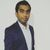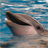# QlikView App Dev

Discussion Board for collaboration related to QlikView App Development.

cancel
Showing results for
Did you mean:Master

## Table monthly

Hi all,

I want to count the numbers  MTD on  strightable

I have a datefield like

Num(Floor(Createdate)) as createDateNum,

how to get MTD for this ? how to write the expression for this?

Thanks

sony

1 Solution

Accepted SolutionsMaster

May be this

sum({<createDateNum = {">=\$(num(monthstart(max(createDateNum))))<=\$(=max(createDateNum) )"}>}Quantity)

5 RepliesCreator II

Please share what you  want calculate from this date field.

Is it monthname of the date .

Share some sample dataCreator

Hi

Try this, if you want to calculate MTD for Sales, Sum({<createDateNum={">=\$(=Num(MonthStart(Max(createDateNum))))<=\$(=Max(createDateNum))"}>} Sales)

Also you can refer to

YTQ, QTD, MTD and WTDMaster

May be this

sum({<createDateNum = {">=\$(num(monthstart(max(createDateNum))))<=\$(=max(createDateNum) )"}>}Quantity)Master
Author

am using same but I am getting the result    jan-05-2018

but MTD means  max month data should come right?  means march?Master